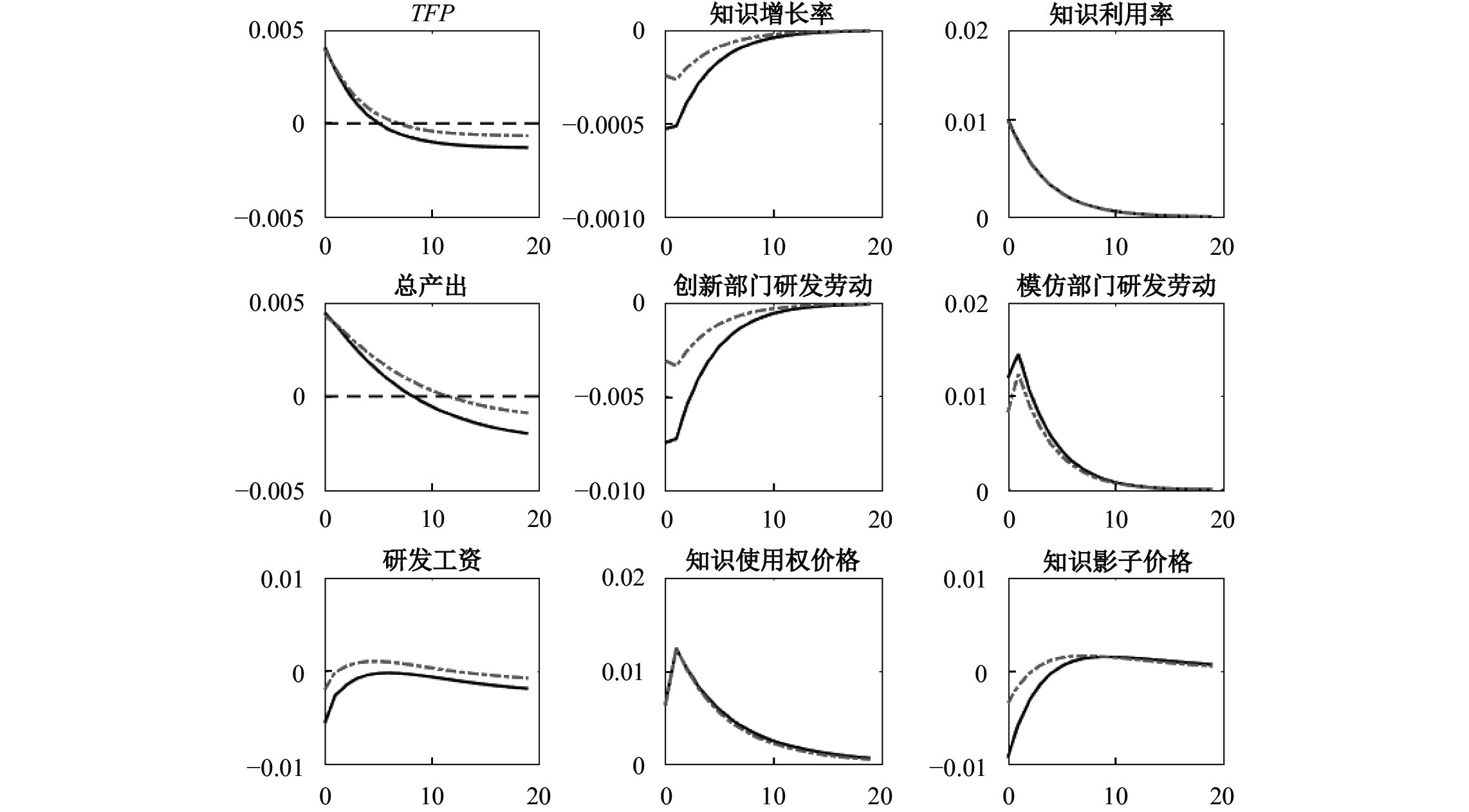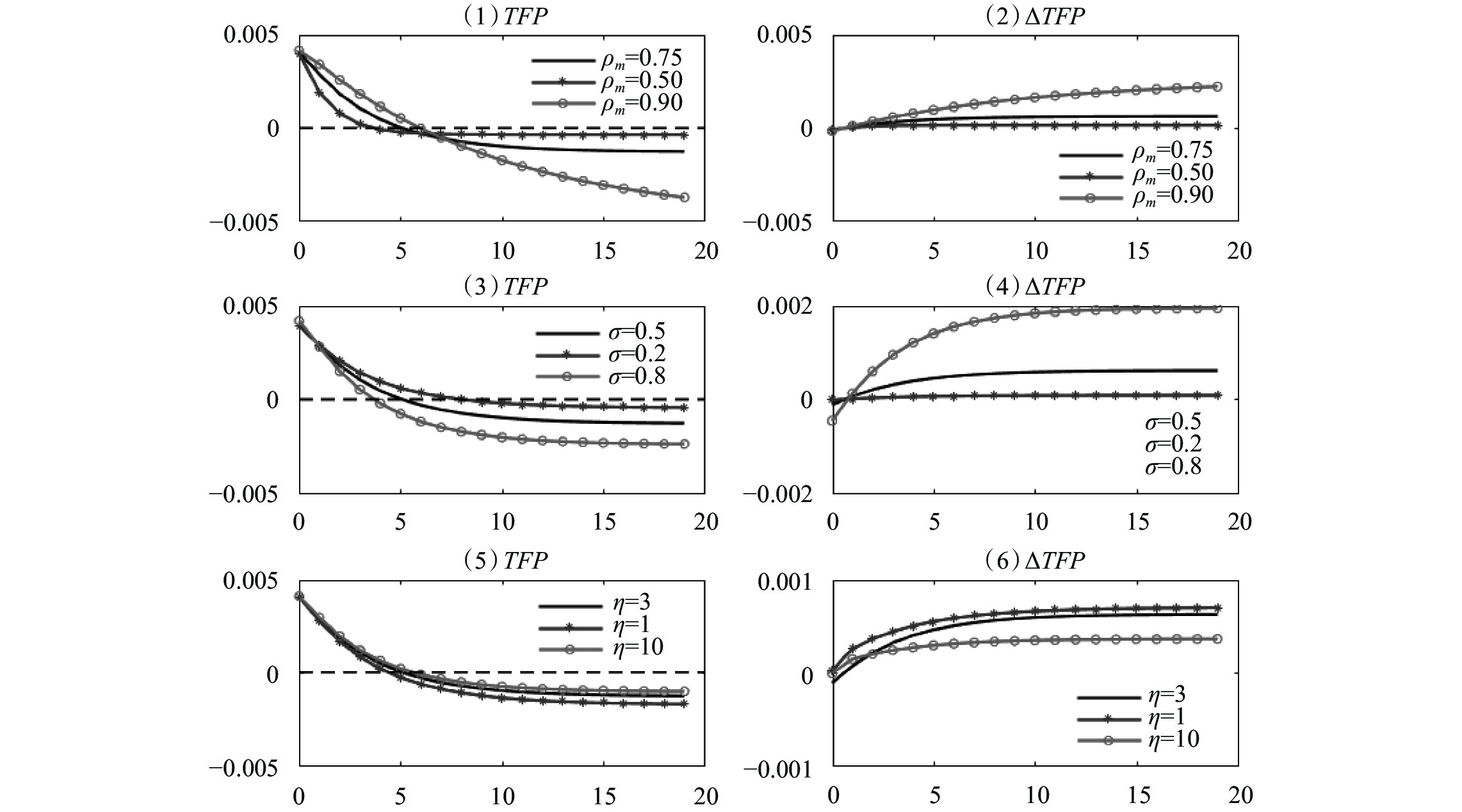﻿ 研发效率、知识产权保护与经济繁荣《财经研究》
2022第48卷第7期

R&D Efficiency, Intellectual Property Protection, and Economic Prosperity
School of Economics，Shanghai University of Finance and Economics，Shanghai 200433，China
Summary: In recent years, representative technologies of the intelligent revolution, such as 5G, big data, and artificial intelligence, have gradually matured and started being applied on a large scale. The advent of the intelligent era implies increase in R&D efficiency of both technology innovation and technology application. From both empirical and theoretical perspectives, this paper explores the macroeconomic impact of R&D efficiency shocks and the role of intellectual property protection (IPP). This paper distinguishes the two types of R&D efficiency — innovation efficiency corresponding to technology innovation and application efficiency corresponding to technology application, and also the two types of firms — innovative firms that focus on knowledge accumulation and imitative firms that focus on knowledge utilization. Using Chinese provincial panel data from 2011 to 2017, the empirical analysis shows that: (1) Increase in innovation efficiency increases the future TFP growth rate, while IPP is not statistically significantly related to this positive effect. (2) Increase in application efficiency decreases the future TFP growth rate, and raising the IPP level weakens this negative effect. This paper then constructs a two-sector DSGE model with endogenous growth. In the model, innovative firms and imitative firms hire R&D labors to accumulate knowledge and to utilize knowledge respectively, and their R&D activities are subject to innovation efficiency shocks and application efficiency shocks respectively. Imitative firms can acquire knowledge of innovative firms in two ways, paying for the right to use knowledge or free access to spillover knowledge, and IPP enables innovative firms to obtain knowledge rents from imitative firms. By simulating the impact of R&D efficiency shocks in two levels of IPP, this paper obtains consistent results with the empirical analysis. Combining the empirical analysis and the theoretical model, this paper introduces the concept of “prosperity trap”, that is, short-term prosperity that overdraws the potential of economic growth, and emphasizes the fact that under positive application efficiency shocks, imitative firms would crowd out innovative firms’ R&D labor, leading to slower knowledge accumulation. Strengthening IPP can avoid the trap by increasing the knowledge rents from imitative firms to innovative firms. This paper has three main contributions: (1) It reveals the macroeconomic impact of R&D efficiency shocks and the heterogeneity, and also highlights the role of IPP under R&D efficiency shocks, while few existing literatures study IPP and R&D efficiency shocks simultaneously. (2) It innovatively introduces the concept of “prosperity trap”, which is an important phenomenon in development economics but has not been focused on as far as our best knowledge. (3) It is an extension for the literature related to DSGE models with endogenous growth, especially for the scarce Chinese literature. Allowing imitative firms to acquire knowledge of innovative firms in two ways is a key theoretical innovation.
Key words: R&D efficiency    intellectual property protection    economic growth potential    DSGE model with endogenous growth

（一）回归方程

 \begin{aligned} dln{TFP}_{i,t+s}= & {\beta }_{0}+{\beta }_{1}{RDgdp}_{i,t}+{\beta }_{2} {TechRgdp}_{i,t}+{\beta }_{3}{RDgdp}_{i,t}\times {IPP}_{i,t} \\ & +{\beta }_{4} {TechRgdp}_{i,t}\times {IPP}_{i,t}+{{\gamma }_{0}}{X}_{i,t}+{\theta }_{t}+{\epsilon }_{i,t+s} \end{aligned}

（二）变量与数据

 $dln{TFP}_{i,t}=dln\left(\frac{{GDP}_{i,t}}{{L}_{i,t}}\right)-0.515\times dln\left(\frac{{K}_{i,t}}{{L}_{i,t}}\right)$

 ${RDgdp}_{it}^{IV}=\frac{{\overline{{RD}}}_{i}}{{\sum }_{i}{\overline{RD}}_{i}}\times \frac{{RD}_{t}}{GD{P}_{t}}$
 ${TechRgdp}_{it}^{IV}=\frac{{\overline{TechR}}_{i}}{{\sum }_{i}{\overline{TechR}}_{i}}\times \frac{{TechR}_{t}}{GD{P}_{t}}$
 ${PhD}_{it}^{IV}=\frac{{\overline{University}}_{i}}{{\sum }_{i}{\overline{University}}_{i}}\times \frac{{PhD}_{t}}{Populatio{n}_{t}}$

（三）回归结果

 （1） OLS （2） IV：2SLS （3） IV：GMM （4） IV：2SLS （5） IV：2SLS （6） IV：2SLS $dlnTF{P}_{i,t+s}$ $(s=3)$ $dlnTF{P}_{i,t+s}$ $(s=3)$ $dlnTF{P}_{i,t+s}$ $(s=3)$ $dlnTF{P}_{i,t+s}$ $(s=2)$ $dlnTF{P}_{i,t+s}$ $(s=4)$ $dlnTF{P}_{i,t+s}$ $(s=-3)$ $RDgdp$ 2.074*** 1.896** 1.749* 2.363*** 1.090 −3.181*** （0.765） （0.903） （1.006） （0.766） （1.324） （0.936） $TechRgdp$ −2.695** −6.431*** −7.001*** −2.940 −6.659*** 1.530 （1.262） （2.401） （2.445） （2.093） （2.442） （2.010） $RDgd\times IPP$ −0.361 −0.463 −0.538 −1.094* 0.339 0.790 （0.416） （0.605） （0.649） （0.556） （0.863） （0.495） $TechRgdp\times IPP$ 1.450** 3.635*** 4.175*** 2.203** 3.580*** −0.257 （0.700） （1.161） （1.159） （1.006） （1.146） （0.921） 观测数 120 120 120 150 90 120 R2 0.408 0.369 0.332 0.358 0.438 0.455 注：***表示 $p < 0.01$ ，**表示 $p < 0.05$ ，*表示 $p < 0.1$ ；括号内为参数估计值的稳健标准差。

（一）代表性家庭

 ${E}_{t}{\sum }_{s=0}^{\infty }{\beta }^{s}\left[\mathrm{l}\mathrm{n}\left({C}_{t+s}\right)-\frac{{\left({\xi }^{L} {L}_{t+s}\right)}^{1+\eta }+{\left({\xi }^{H} {H}_{t+s}\right)}^{1+\eta }}{1+\eta }\right]$

 ${C}_{t}+\frac{{B}_{t+1}}{{r}_{t}}={W}_{t}^{L}{L}_{t}+{W}_{t}^{H}{H}_{t}+{B}_{t}+{D}_{t}$

（二）中间产品企业和知识服务商

 ${Y}_{n,t}={\int }_{0}^{1}{Y}_{n,j,t}dj,\;\;{Y}_{m,t}={\int }_{0}^{1}{Y}_{m,j,t}dj$

 ${Y}_{i,j,t}={\left({K}_{i,j,t}\right)}^{\alpha }{\left({A}_{i,j,t}{L}_{i,j,t}\right)}^{1-\alpha }$

 ${A}_{i,j,t}={u}_{i,j,t}{N}_{i,j,t}$

 ${K}_{i,j,t+1}=\left(1-{\delta }_{K}\right){K}_{i,j,t}+{I}_{i,j,t}$

1. 创新型企业。创新型企业 $j\in [0, 1]$ 自行积累知识存量，假设其知识存量可以完全利用，知识利用率 ${u}_{n,j,t}\equiv 1$ ，且无需支付额外的知识利用成本。

 ${N}_{n,j,t+1}=\left(1-{\delta }_{N}\right){N}_{n,j,t}+{\chi }_{n,t}{N}_{n,t}{\left({H}_{n,j,t}\right)}^{\varrho }$ (1)

 ${P}_{n,j,t}{Y}_{n,j,t}+{P}_{N,j,t}{N}_{n,j,t}={W}_{t}^{L}{L}_{n,j,t}+{W}_{t}^{H}{H}_{n,j,t}+{I}_{n,j,t}+{D}_{n,j,t}$

 ${E}_{t}\left\{\beta \frac{{C}_{t}}{{C}_{t+1}}\left[\left(1-{\delta }_{N}\right){Q}_{N,t+1}+\frac{\left(1-\mathrm{\alpha }\right) {P}_{n,t+1}{Y}_{n,t+1}}{{N}_{n,t+1}}+{P}_{N,n,t+1}\right]\right\}={Q}_{N,t}$ (2)
 ${Q}_{N,t}\left[{\chi }_{n,t}{N}_{n,t} \varrho {\left({H}_{n,t}\right)}^{\varrho -1}\right]={W}_{t}^{H}$ (3)

2. 知识服务商。知识服务商每期向创新型企业 $j$ 支付知识租金 ${P}_{N,j,t}{N}_{n,j,t}$ 以获得知识使用权，并对知识和知识使用权进行打包，打包的知识为 ${N}_{n,t}={\int }_{0}^{1}{N}_{n,j,t}dj$ 。假设在知识服务商打包的过程中， $1-\omega \in \left(\mathrm{0,1}\right)$ 比例的知识发生溢出，被模仿型企业免费获得。知识服务商将剩余未溢出知识 $\omega {N}_{n,t}$ 的使用权按照每单位知识单期的价格 ${P}_{N,t}$ 出售给模仿型企业。由利润最大化和零利润可得：

 ${P}_{N,n,t}=\omega {P}_{N,t}$ (4)

3. 模仿型企业。模仿型企业 $j\in [0, 1]$ 自身不积累知识，但可以通过两种方式获得创新型企业所积累的知识：一是免费获得溢出的知识 $(1-\omega ){N}_{n,t}$ ；二是向知识服务商购买知识使用权 ${\overline{N}}_{m,j,t}$ ，单位知识使用权的购买价格为 ${P}_{N,t}$ 。因此，模仿型企业 $j$ 可使用的知识存量为：

 ${N}_{m,j,t}={\overline{N}}_{m,j,t}+(1-\omega ){N}_{n,t}$

 ${u}_{m,j,t}={\frac{1}{\gamma }{\chi }_{m,t}\left({H}_{m,j,t}\right)^{\gamma }}$ (5)

 ${P}_{m,j,t}{Y}_{m,j,t}={W}_{t}^{L}{L}_{m,j,t}+{W}_{t}^{H}{H}_{m,j,t}+{P}_{N,t}{\overline{N}}_{m,j,t}+{I}_{m,j,t}+{D}_{m,j,t}$

 $\left(1-\mathrm{\alpha }\right) \frac{{P}_{m,t}{Y}_{m,t}}{{N}_{n,t}}={P}_{N,t}$ (6)
 $\frac{\gamma \left(1-\alpha \right){P}_{m,t}{Y}_{m,t}}{{H}_{m,t}}={W}_{t}^{H}$ (7)

（三）最终产品企业

 ${Y}_{t}={\left(\varphi {Y}_{n,t}^{(\sigma -1)/\sigma }+\left(1-\varphi \right){Y}_{m,t}^{(\sigma -1)/\sigma }\right)}^{\sigma /(\sigma -1)}$

 ${Y}_{n,t}={\varphi }^{\sigma }{\left(\frac{{P}_{n,t}}{{P}_{t}}\right)}^{-\sigma } {Y}_{t},\;\;{Y}_{m,t}={\left(1-\varphi \right)}^{\sigma }{\left(\frac{{P}_{m,t}}{{P}_{t}}\right)}^{-\sigma } {Y}_{t}$
 ${P}_{t}={\left({\varphi }^{\sigma }{P}_{n,t}^{1-\sigma }+{\left(1-\varphi \right)}^{\sigma }{P}_{m,t}^{1-\sigma }\right)}^{1/(1-\sigma )}$

（四）市场出清及其他

 ${TFP}_{t}=\frac{{Y}_{t}}{{\left({K}_{n,t}+{K}_{m,t}\right)}^{\alpha } {L}_{t}^{1-\alpha }}$

（五）趋势变量与知识增长率

 ${\mu }_{n,t}=\left(1-{\delta }_{N}\right)+{\chi }_{n,t} {\left({H}_{n,t}\right)}^{\varrho }$ (8)

（一）参数校准

（二）结果分析

1. 创新效率冲击。创新效率冲击的脉冲响应曲线如图1所示，其中实线表示基准保护水平（ $\omega =0.003$ ），虚线表示严格保护水平（ $\omega =0.2$ ）。在基准保护水平下，正向的创新效率冲击导致创新型企业单位研发劳动投入所产生的新知识增加，鼓励创新型企业增加研发劳动投入 ${H}_{n,t}$ ，促使知识增长率 ${\mu }_{n,t}$ 上升。创新型企业研发劳动投入的增加和知识增长率的上升共同促使研发工资上升。知识存量的加速增长提高了中长期TFP和总产出水平，也使创新部门产出上升。知识存量的加速增长还使知识使用权的供给增加，知识的边际产出下降，从而降低了知识使用权价格和知识影子价格。此外，创新型企业研发劳动的增加挤出了模仿型企业的研发劳动投入 ${H}_{m,t}$ ，降低了其知识利用率 ${u}_{m,t}$ ，导致短期内其产出下降，TFP和总产出也下降，对经济造成负面影响。而从中长期看，模仿型企业可使用的知识存量因社会知识总量上升而增加，所以其产出仍会增长。图 1 创新效率冲击的脉冲响应分析 注：创新效率冲击对重要变量的影响（${\rho }_{n}=0.75$，${\sigma }_{n}=0.01$）。横坐标为时期，纵坐标为变量偏离初始稳态的百分比。

2. 应用效率冲击。应用效率冲击的脉冲响应曲线如图2所示。同样地，实线表示基准保护水平（ $\omega =0.003$ ），虚线表示严格保护水平（ $\omega =0.2$ ）。在基准保护水平下，正向的应用效率冲击提高了模仿型企业的知识利用率 ${u}_{m,t}$ ，并鼓励模仿型企业投入更多的研发劳动 ${H}_{m,t}$ ，从而对模仿部门产出、总产出和TFP具有短期的正面影响。然而，模仿型企业研发劳动投入的增加挤出了创新型企业的研发劳动投入 ${H}_{n,t}$ ，降低了知识积累速度 ${\mu }_{n,t}$ ，从而减少了创新部门的产出和知识使用权的供给。模仿型企业知识利用率的上升提高了知识使用权的边际产出，所以知识使用权的需求增加。供给减少和需求增加共同导致知识使用权价格上升，增加了创新型企业单位知识存量的知识租金，形成了正向反馈作用。图 2 应用效率冲击的脉冲响应分析 注：应用效率冲击对重要变量的影响（${\rho }_{m}=0.75$，${\sigma }_{m}=0.01$）。横坐标为时期，纵坐标为变量偏离初始稳态的百分比。

（三）稳健性讨论图 3 不同参数下应用效率冲击对TFP的影响和知识产权保护的作用 注：应用效率冲击对TFP的影响以及知识产权保护对TFP变化幅度的影响（${\sigma }_{m}=0.01$）。横坐标为时期，纵坐标为变量偏离初始稳态的百分比。（1）、（3）和（5）表示重新校准后知识产权保护基准水平下的TFP变化，（2）、（4）和（6）表示重新校准后两种知识产权保护水平下TFP变化幅度的差值。（1）和（2）展示应用效率冲击持续性参数${\rho }_{m}$的影响，（3）和（4）展示知识积累中拥塞效应系数$\varrho$的影响，（5）和（6）展示Frisch劳动供给弹性的倒数$\eta$的影响。

① 吴超鹏和唐菂（2016）的实证结果表明，减少研发溢出是知识产权保护促进企业创新的重要渠道。

② 基于中国2012—2019年工业企业数据，R&D人员折合全时当量约为总就业人数的0.5%，由此推算创新部门研发劳动 ${H}_{n}$ 为0.002。基于模型假设和数据定义，可将规模以上工业企业的R&D经费支出与模型中创新型企业的研发劳动工资支出相对应，将技术改造经费支出与模型中模仿型企业的研发劳动工资支出相对应。由于数据中规模以上工业企业技术改造经费支出与R&D经费支出的比值约为36%，模仿部门研发劳动 ${H}_{m}$ 设为 ${0.36H}_{n}$ 。综上，社会总研发劳动等于两个部门研发劳动之和 $H={H}_{n}+{H}_{m}=0.00272$

③ 张勋和乔坤元（2016）利用1985—2013年中国省级面板数据和空间计量方法，估计得到各经济圈的技术扩散参数，本文中模仿型企业的付费知识占比 $\omega$ 的校准值在其估计结果区间[0.001，0.020]内。

④ 重新校准时，基准知识产权保护水平下创新型企业的知识租金收入与研发费用的稳态比值为2.6%，严格知识产权保护水平下创新型企业的知识租金收入与研发费用的稳态比值为1。对 ${\rho }_{m}$ 的重新校准不会改变知识产权保护水平 $\omega$ 的值，但对 $\varrho$ $\eta$ 的重新校准会改变 $\omega$ 的值。

  陈凤仙, 王琛伟. 从模仿到创新——中国创新型国家建设中的最优知识产权保护[J]. 财贸经济, 2015(1): 143–156. DOI:10.3969/j.issn.1005-913X.2015.01.072  陈凯华, 汪寿阳, 寇明婷. 三阶段组合效率测度模型与技术研发效率测度[J]. 管理科学学报, 2015(3): 31–44.  戴魁早, 刘友金. 要素市场扭曲与创新效率——对中国高技术产业发展的经验分析[J]. 经济研究, 2016(7): 72–86.  官建成, 陈凯华. 我国高技术产业技术创新效率的测度[J]. 数量经济技术经济研究, 2009(10): 19–33.  郭春野, 庄子银. 知识产权保护与“南方”国家的自主创新激励[J]. 经济研究, 2012(9): 32–45.  韩先锋, 惠宁, 宋文飞. 贸易自由化影响了研发创新效率吗?[J]. 财经研究, 2015(2): 15–26.  姜军, 江轩宇, 伊志宏. 企业创新效率研究——来自股权质押的影响[J]. 金融研究, 2020(2): 128–146.  金相郁. 中国区域全要素生产率与决定因素: 1996-2003[J]. 经济评论, 2007(5): 107–112.  刘小鲁. 知识产权保护、自主研发比重与后发国家的技术进步[J]. 管理世界, 2011(10): 10–19.  潘健平, 潘越, 马奕涵. 以“合”为贵? 合作文化与企业创新[J]. 金融研究, 2019(1): 148–167.  庞瑞芝, 杨慧. 中国省际全要素生产率差异及经济增长模式的经验分析——对30个省（市、自治区）的实证考察[J]. 经济评论, 2008(6): 16–22.  魏浩, 巫俊. 知识产权保护、进口贸易与创新型领军企业创新[J]. 金融研究, 2018(9): 91–106.  吴超鹏, 唐菂. 知识产权保护执法力度、技术创新与企业绩效——来自中国上市公司的证据[J]. 经济研究, 2016(11): 125–139.  肖文, 林高榜. 政府支持、研发管理与技术创新效率——基于中国工业行业的实证分析[J]. 管理世界, 2014(4): 71–80.  熊曦, 关忠诚, 杨国梁, 等. 嵌套并联结构两阶段DEA下科技创新效率测度与分解[J]. 中国管理科学, 2019(3): 206–216.  徐舒, 左萌, 姜凌. 技术扩散、内生技术转化与中国经济波动——一个动态随机一般均衡模型[J]. 管理世界, 2011(3): 22–31.  杨轶波. 增强知识产权保护总能促进创新吗? ——纳入“干中学”效应的南北框架分析[J]. 世界经济研究, 2018(12): 115–131.  张军, 吴桂英, 张吉鹏. 中国省际物质资本存量估算: 1952-2000[J]. 经济研究, 2004(10): 35–44.  张勋, 乔坤元. 中国区域间经济互动的来源: 知识溢出还是技术扩散?[J]. 经济学（季刊）, 2016(4): 1629–1652.  赵甜, 方慧. OFDI与中国创新效率的实证研究[J]. 数量经济技术经济研究, 2019(10): 58–76.  钟昀珈, 张晨宇, 陈德球. 国企民营化与企业创新效率: 促进还是抑制?[J]. 财经研究, 2016(7): 4–15.  朱东平. 外商直接投资、知识产权保护与发展中国家的社会福利——兼论发展中国家的引资战略[J]. 经济研究, 2004(1): 93–101.  朱有为, 徐康宁. 中国高技术产业研发效率的实证研究[J]. 中国工业经济, 2006(11): 38–45.  Anzoategui D, Comin D, Gertler M, et al. Endogenous technology adoption and R&D as sources of business cycle persistence[J]. American Economic Journal: Macroeconomics, 2019, 11(3): 67–110. DOI:10.1257/mac.20170269  Bianchi F, Kung H, Morales G. Growth, slowdowns, and recoveries[J]. Journal of Monetary Economics, 2019, 101: 47–63. DOI:10.1016/j.jmoneco.2018.07.001  Chang C, Chen K J, Waggoner D F, et al. Trends and cycles in China’s macroeconomy[J]. NBER Macroeconomics Annual, 2016, 30(1): 1–84. DOI:10.1086/685949  Chang C, Liu Z, Spiegel M M. Capital controls and optimal Chinese monetary policy[J]. Journal of Monetary Econo- mics, 2015, 74: 1–15. DOI:10.1016/j.jmoneco.2015.04.003  Comin D, Gertler M. Medium-term business cycles[J]. American Economic Review, 2006, 96(3): 523–551. DOI:10.1257/aer.96.3.523  Comin D, Loayza N, Pasha F, et al. Medium term business cycles in developing countries[J]. American Economic Journal: Macroeconomics, 2014, 6(4): 209–245. DOI:10.1257/mac.6.4.209  Griliches Z. Patent statistics as economic indicators: A survey[J]. Journal of Economic Literature, 1990, 28(4): 1661–1707.  Grossman G, Helpman E. Endogenous product cycles[J]. Economic Journal, 1991, 101(408): 1214–1229. DOI:10.2307/2234437  Helpman E. Innovation, imitation, and intellectual property rights[J]. Econometrica, 1993, 61(6): 1247–1280. DOI:10.2307/2951642  Kung H, Schmid L. Innovation, growth, and asset prices[J]. The Journal of Finance, 2015, 70(3): 1001–1037. DOI:10.1111/jofi.12241  Lai E L C. International intellectual property rights protection and the rate of product innovation[J]. Journal of Development Economics, 1998, 55(1): 133–153. DOI:10.1016/S0304-3878(97)00059-X  Moran P, Queralto A. Innovation, productivity, and monetary policy[J]. Journal of Monetary Economics, 2018, 93: 24–41. DOI:10.1016/j.jmoneco.2017.10.006  Queralto A. A model of slow recoveries from financial crises[J]. Journal of Monetary Economics, 2020, 114: 1–25. DOI:10.1016/j.jmoneco.2019.03.008